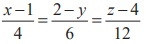Home | | Maths 12th Std | Angle between two straight lines

# Angle between two straight lines

Mathematics : Applications of Vector Algebra: Application of Vectors to 3-Dimensional Geometry: Angle between two straight lines

## Angle between two straight lines

### (a) Vector form

The acute angle between two given straight lines### (b) Cartesian form

If two lines are given in Cartesian form asthen the acute angle θ between the two given lines is given byRemark

(i) The two given lines with direction ratios b1 , b2 , band d1 , d2 , dare parallel if, and only if.

(ii)  The two given lines with direction ratios b1, b2, b3 and d1, d2, d3 are perpendicular if and only if b1d1 + b2d2 + b3d3 = 0 .

(iii) If the direction cosines of two given straight lines are l1 , m1 , n1 and l2 , m2 , n2, then the angle between the two given straight lines is cos θ =| l1l2 + m1m2 + n1n2 | .

### Example 6.29

Find the acute angle between the lines= (ˆ+ 2ˆ+ 4ˆ) + t(2ˆ+ 2ˆˆ) and the straight line passing through the points (5,1, 4) and (9, 2,12) .

### Solution

We know that the line= (ˆ+ 2ˆ+ 4ˆ) + t(2ˆ+ 2ˆˆ) is parallel to the vector 2ˆ+ 2ˆˆk.

Direction ratios of the straight line joining the two given points (5,1, 4) and (9, 2,12) are 4,1,8 and hence this line is parallel to the vector 4iˆ + ˆ+ 8kˆ .

Therefore, the acute angle between the given two straight lines is### Example 6.30

Find the acute angle between the straight linesand state whether they are parallel or perpendicular.

### Solution

Comparing the given lines with the general Cartesian equations of straight lines,we find (b1 , b2 , b3 ) = (2,1, −2) and (d1 , d2 , d3 ) = (4, −4, 2) . Therefore, the acute angle between the two straight lines isThus the two straight lines are perpendicular.

### Example 6.31

Show that the straight line passing through the points A(6, 7, 5) and B(8,10, 6) is perpendicular to the straight line passing through the points C(10, 2, −5) and D(8, 3, −4) .

### Solution

The straight line passing through the points A(6, 7, 5)  and B(8,10, 6) is parallel to the vector−= 2iˆ + 3 ˆkˆ and the straight line passing through the points C(10, 2, −5) and D(8, 3, −4) is parallel to the vector= −2iˆ + ˆkˆ . Therefore, the angle between the two straight lines is the angle between the two vectorsand. Sincethe two vectors are perpendicular, and hence the two straight lines are perpendicular.

Aliter

We find that direction ratios of the straight line joining the points A(6, 7, 5) and B(8,10, 6) are (b1 , b2 , b3 ) = (2, 3,1) and and direction ratios of the line joining the points C(10, 2, −5) and D(8, 3, −4) are (d1 , d2 , d3 ) = (−2,1,1) . Since b1d1 + b2d2 + b3d3 = (2)(−2) + (3)(1) + (1)(1) = 0 , the two straight lines are perpendicular.

Example 6.32

Show that the linesandare parallel

Solution

We observe that the straight lineis parallel to the vector 4iˆ - 6 ˆj +12kˆ and the straight lineis parallel to the vector -2iˆ + 3ˆj - 6kˆ.

Since 4iˆ - 6ˆj +12kˆ = -2(-2iˆ + 3ˆj - 6kˆ) , the two vectors are parallel, and hence the two straight lines are parallel.

Tags : Definition, Theorem, Proof, Solved Example Problems, Solution , 12th Mathematics : UNIT 6 : Applications of Vector Algebra
Study Material, Lecturing Notes, Assignment, Reference, Wiki description explanation, brief detail
12th Mathematics : UNIT 6 : Applications of Vector Algebra : Angle between two straight lines | Definition, Theorem, Proof, Solved Example Problems, Solution